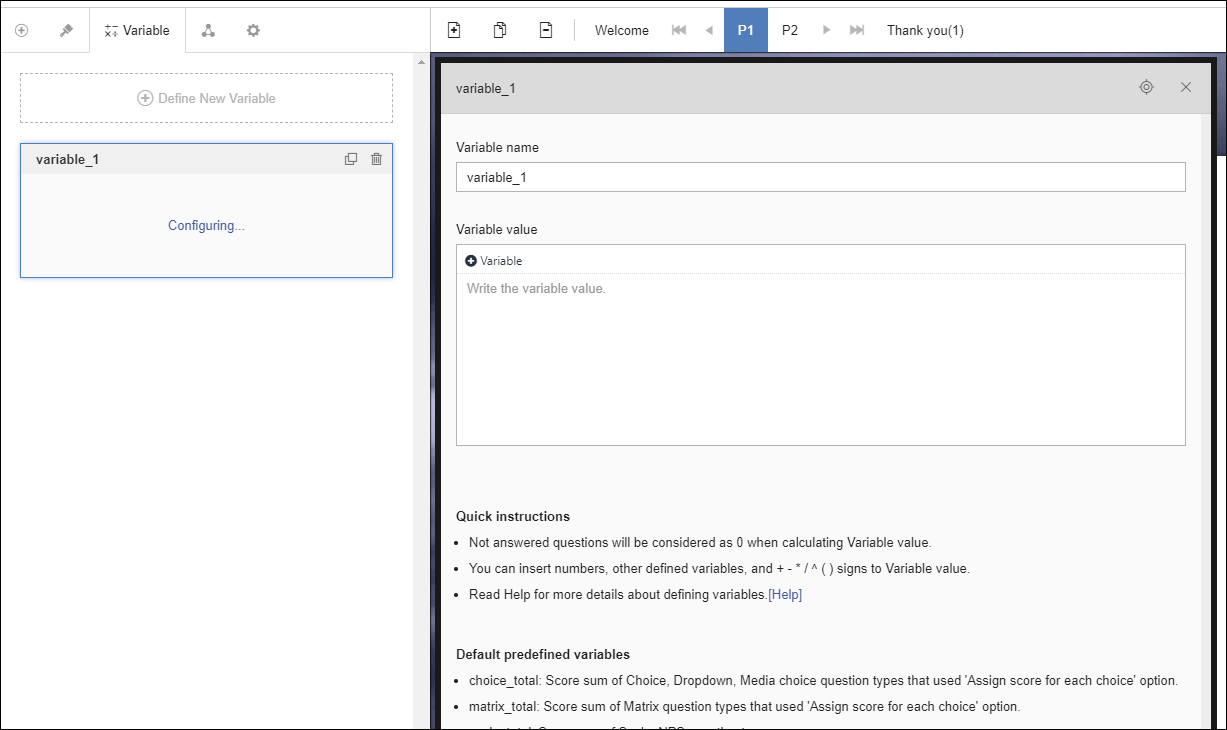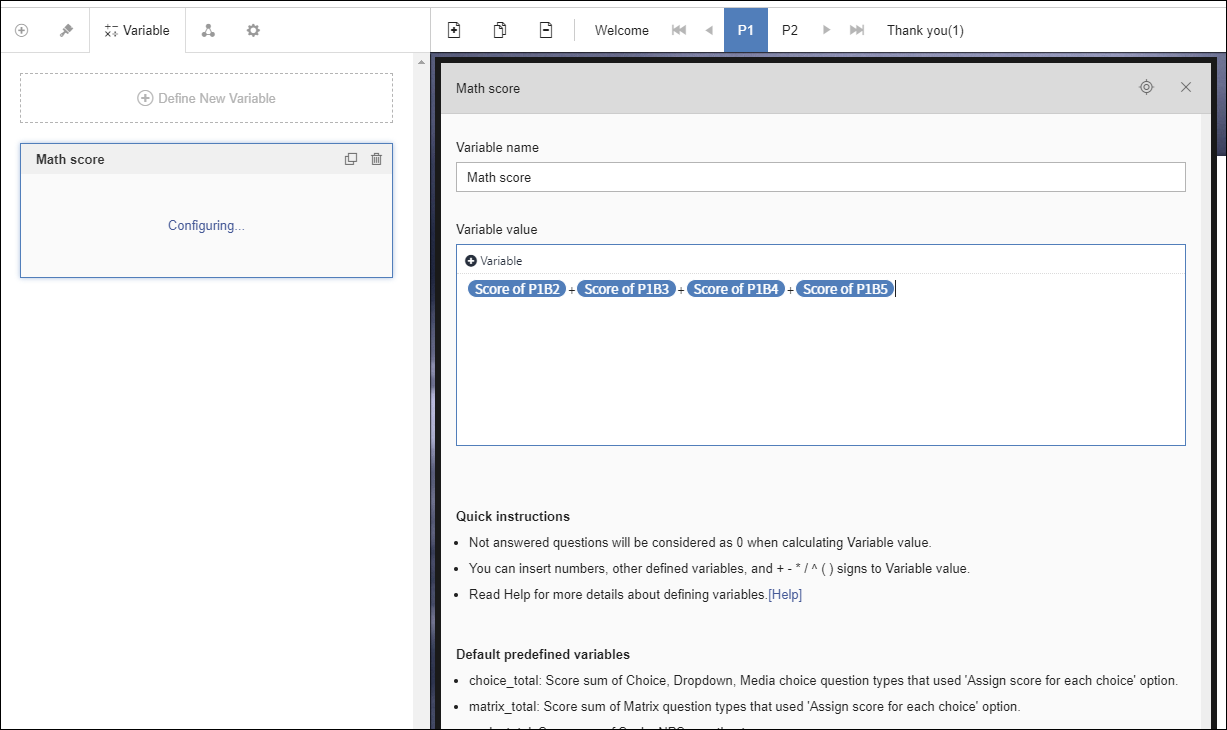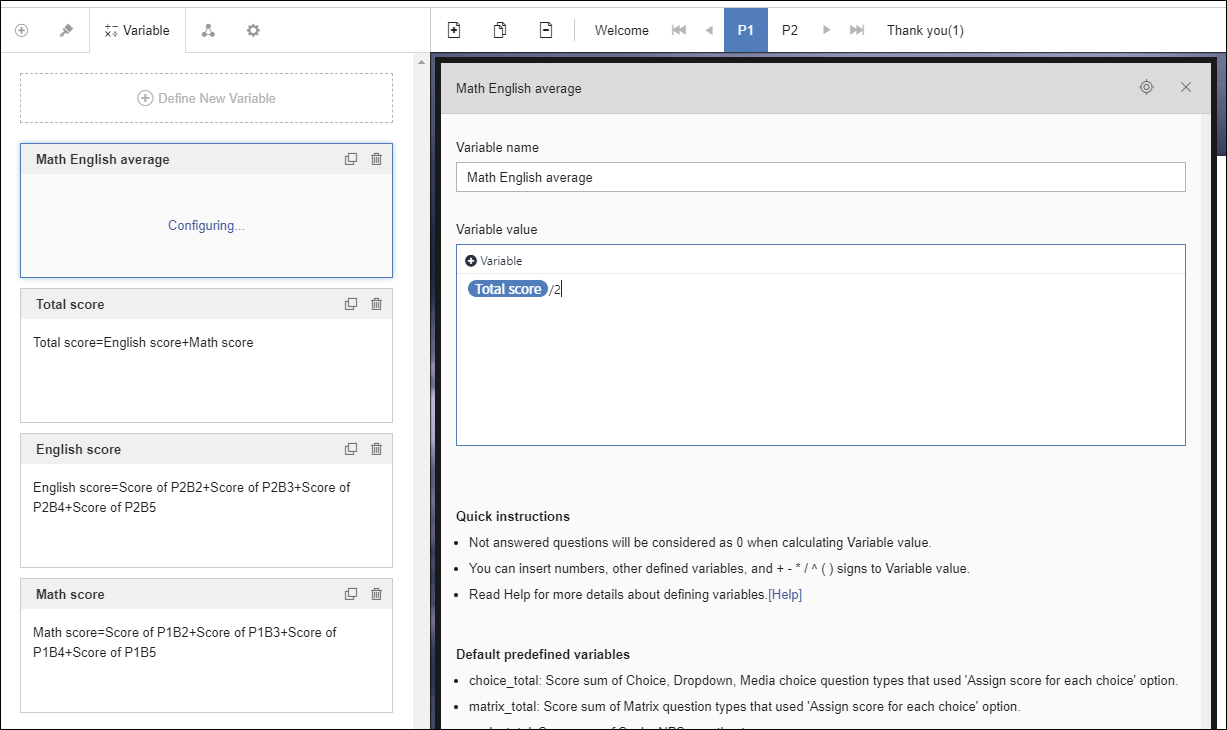# Defined Variable

You can define your own variables and insert them into the form or use them in logic.

For example, you can create 4 math questions, assign a score to each question, define the sum of the scores of the 4 questions as a variable called 'Math score', and insert this variable in the questionnaire to show the respondent the score. You can also set Show/Hide, Jump to, and End survey logic according to the score of this variable by using the ‘Math score’ variable as a logic condition.

### How to set

You can define a variable by going to the ‘Variable’ tab at the top of the left panel of the form editing screen and clicking the ‘Define New Variable’ button.You can write the name of the variable you want in ‘Variable name’, and write the formula in ‘Variable value’. In the ‘Variable value’ input window, click the ‘+ Variable’ button to insert a score variable and configure the calculation formula using the operation symbols.In the 'Variable value' input window, you can input score variables for questions with scores, answer variables for numerical open-ended questions, other defined variables, numbers and operation symbols (+, -, *, /, (, ), ^).Tip!

You can insert a choice item such as P1B1C1 in the variable value. A choice item gets the score assigned to that choice only when that choice is selected as an answer. If that choice is not selected, the item is calculated as 0.

### Predefined variables

There are predefined variables in addition to the variables defined by the user in the above method.

• choice_total: The sum of the scores of all choice questions in this form. (Single choice, Multiple choice, Dropdown, Media single choice, Media multiple choice)
• matrix_total: The sum of the scores of all Matrix questions in this form. (Matrix single choice, Matrix multiple choice)
• scale_total: The sum of the scores of all scale and NPS questions in this form.
• rating_total: The sum of the scores of all rating questions in this form.

Caution

As the predefined variables are calculated as the sum of the scores for all questions in the form, the result value can be changed until respondents answer the last question, so please be careful when inserting it into logic or the form.

### Error cases

The cases where a defined variable is recognized as an error are as follows.

• Incorrect operation symbol input when inputting variable value: Especially when multiple parentheses are used, the pair of opening and closing parentheses must match.
• Errored or deleted variables within variable value: If the inserted variable within variable value has been deleted or changed to a non-calculable item, or if the scoring option is later turned off, it is an error because it cannot be properly calculated.

In the above cases, the corresponding variable is displayed as an error in the variable list, and all logics including these variables are treated as error logic and do not work.

Even though the defined variable itself is not an error, when the calculation result is mathematically error (eg, when a number is divided by 0) or when the result value is greater than 999,999,999,999,999, it is recognized as an error, and the logic condition with the variable is not evaluated and is also marked as an error in the form.

Caution

If the variable itself is an error, the logic containing the variable also becomes an error and the entire logic does not work. If only the result value of a variable is an error, only the condition containing the variable of the logic becomes an error and only that condition is not evaluated.

### Range of calculation result

As mentioned above, the calculation result of the defined variable must be less than 999,999,999,999,999, and it is displayed to 5 decimal places.

Caution

• You must set WHEN setting manually when making Jump to logic and End survey logic that are conditional on defined variables.
• When calculating variable values, all unanswered items are counted as 0.
• When inputting a variable value, be sure to put an operation symbol between the items. Do not omit the operator, such as 2x or 3 (2+5).
• Variable names can be set to be duplicated with other variable names, but they can be confusing when using them, so please use them separately if possible.